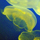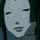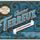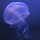## General Question# How can I obtain the integral equation f(x)?

Asked by xichyu (222) September 28th, 2016

The derivative of f(x) is equal to the square root of f(x)，and the initial condition is:
f(x)=0，
How can I obtain f(x)?

Observing members: 0Composing members: 0Response moderated@xichyu look up your exponent rules involving square roots and then remember the power rule from way back when in calc I. This is literally the easiest calc problem ever.

ARE_you_kidding_me (19773)“Great Answer” (1) Flag as…@xichyu do you mean f(0) = 0?

cinnamonk (5397)“Great Answer” (1) Flag as…To get f(x), just perform integration on both sides of the equation. You get f(x) equals to integrated square root of f(x), where f(0) is 0.

citizenearth (781)“Great Answer” (0) Flag as…or